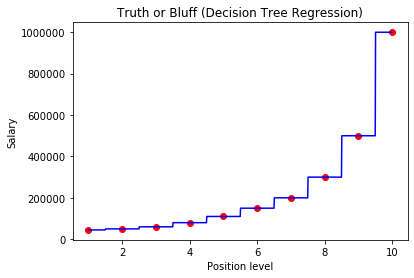0%

## Decision Tree Regression

A decision tree is a flowchart-like method. Each internal node in a decision tree model adresses or represents a "result|outcome" of an attribute (e.g. whether a dice roll comes up with a 1 or a 6), each branch represents the outcome of the test, and each leaf node represents a class label (decision taken after computing all attributes)

Here is how a decision tree looks like:For this model we will be using decision tree to predict a salary of an employee with position level of 6.5

## Lets Start

In :
# Importing the libraries
import numpy as np
import matplotlib.pyplot as plt
import pandas as pd


## Create my Own Dataset

In :
df = pd.DataFrame({'Position': ['Business Analyst', 'Junior Consultant', 'Senior Consultant', 'Manager', 'Country Manager', 'Region Manager', 'Partner', 'Senior Partner', 'c-level', 'CEO'], 'Level':[1,2,3,4,5,6,7,8,9,10], 'Salary': [45000, 50000, 60000, 80000, 110000, 150000, 200000, 300000, 500000, 1000000]})
x = df.iloc[: , 1:2].values
y = df.iloc[:, 2:3].values
print(df)

            Position  Level   Salary
1  Junior Consultant      2    50000
2  Senior Consultant      3    60000
3            Manager      4    80000
4    Country Manager      5   110000
5     Region Manager      6   150000
6            Partner      7   200000
7     Senior Partner      8   300000
8            c-level      9   500000
9                CEO     10  1000000

In :
print(x.T, '\n',y.T)

[[ 1  2  3  4  5  6  7  8  9 10]]
[[  45000   50000   60000   80000  110000  150000  200000  300000  500000
1000000]]


## Fitting Decision Tree Regression to the dataset

In :
from sklearn.tree import DecisionTreeRegressor
regressor = DecisionTreeRegressor(random_state = 0)
regressor.fit(x, y)

# Predicting a new result
y_pred = regressor.predict([[7.5]])


## Visualising the Decision Tree Regression results (higher resolution)

In :
X_bar = np.arange(min(x), max(x), 0.01)
X_bar = X_bar.reshape((len(X_bar), 1))
plt.scatter(x, y, color = 'red')
plt.plot(X_bar, regressor.predict(X_bar), color = 'blue')
plt.title('Truth or Bluff (Decision Tree Regression)')
plt.xlabel('Position level')
plt.ylabel('Salary')
plt.show()